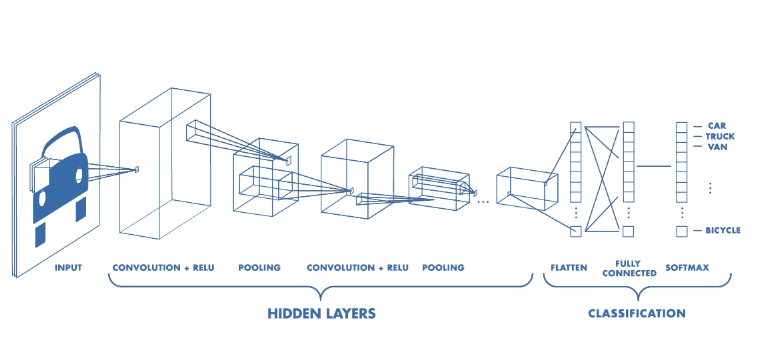# Convolution Neural Network?

Original article can be found here (source): Deep Learning on Medium# Convolution Neural Network?

Welcome everyone! This is my sixth writing on my journey of Completing the Deep Learning Nanodegree in a month! I’ve done 25% of the third module out of a total of six modules of the degree. Today’s topic was Convolutional Neural Networks, CNNs.

## Day- 7

I finished the Lesson of CNNs today and I noted some key points.

## Need of CNNs

We have done MLPs till now and yeah, it does a fine job on training models on the MNIST data, only I’ve dealt with till now. But when we talk about models which perform tasks like image processing, or the models that drive a self-drive car, or perhaps our face recognition systems, then MLPs don’t do such a good job. The thing is that with MLPs, we can’t really generalize a model because the model would fail to classify an upside down image, or an image that is tilted a bit to its right, etc. This shows that the MLPs are not so good for Images. What now? We use Convolutional Neural Networks.

## Main difference

The main difference between CNNs and MLPs is that MLPs require vectors as inputs and when we have to deal with images, we flatten he image pixels into a 1D vector and then pass it. What we don’t realize is that during flattening the image, we lose some valuable information about the image which later would have been useful in classifying the inputs. On the other hand, CNNs do not require vectors as inputs, rather they accept whole matrices. Hence why, we can input a picture, because after all the image is just a 2D array of its RGB values and the fact that it inputs a whole matrix can in itself become the prime reason to choose CNNs over MLPs while having image inputs.

## Data

Lets talk about the normalization process while using CNNs. For preparing the data to be used in a CNN, we normalize the data in the following manner:

`# Normalizing DataData = (value - mean(data))/std(data)`

Next is, the data parts. The data now is divided into three parts train, test & validation sets. Lets recall the process fo avoiding Overfitting. We calculated the training loss and the validation loss and until the time when both of them decreased, the model was improving but once the validation loss stops decreasing and the train loss continues to do so, that point is where the model starts to overfit.

Model Validations give the answer to the important question that when to Stop Training!

# CNN Process

Lets talk about how a Convolutional Neural Network works.

The main difference here, is the way to analyze the data to find underlying information and relations between the features. In this, we divide the image into parts and give the parts one by one to each of the hidden layer nodes. Each of the nodes then have to compute the results of the part of the image they are assigned to and not the full image as before. In this way, we can find more patterns un the different parts of the images and then combine them to form an algorithm. And in this way, the layers have lesser parameters they have to work on which makes their jobs a bit faster.

We group the hidden nodes to select a feature from the input and then each of the node in the group gets assigned a different part of the input and then works its way to do the job. In this way, we will have groups of nodes with each node representing a specific place in the input and each node group for different feature. We can use similar weights in each of the hidden node group because they can have similar information to test.

Any Pattern that can be relevant in understanding the image can be anywhere in the image.

## Convolutional Kernels

These are grids of matrices used to change an image. These, when multiplied with the image data, yields important information about that part of the image. These help in finding patterns like Edges, Background & Foreground, etc. For example, when we need to find edges in a picture, then the matrix must have elements such that there sum is equal to zero and these matrices are often called ‘Weights’. We take the picture which we want to analyze, get some pixels out and then multiply the weights matrix and then sum the values and the number that is returned tells us the about the edge.

High Pass Filters are used to make an image sharper and to enhance high frequency parts of an image. Initializing is as follows. Please note that stride and padding will be covered later.

Edges are areas in the image where the intensity of the images changes rapidly and it often.

`self.conv = nn.Conv2d(input_layer_depth, output_layer_depth, size_of_the_image, stride, padding)`

## Weight Matrix

What happens is that we map a new matrix to the image and store some kind of useful information out of the previous layer.